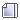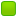###Author Topic: Question 3 in Calculus Proof Questions  (Read 11077 times)

0 Members and 1 Guest are viewing this topic.

####Ender

• Moderator
• Hero Member
••• Posts: 2398##### Re: Question 3 in Calculus Proof Questions
« Reply #15 on: May 25, 2007, 02:23:51 pm »
But since it says in the question that lim x -> 0 f'(x) exists, doesn't that imply the lefthand and righthand limits agree? This rules out jump discontinuities. Also, as infinity as a limit does not make the limit exist -- since limits only exist as numbers -- this rules out infinite discontinuities as well. So we've ruled out removable discontinuities, jump discontinuities, and infinite discontinuities,  thus there can be no discontinuity at x = 0 since there are no other types of discontinuities left for all that single var. calc is concerned.

I guess I should include this in my proof to complete it, but I'll wait for you to respond.

By the way, this question seems to be rooted in analysis, albeit basic analysis.
« Last Edit: May 25, 2007, 02:56:46 pm by Ender »

####Rule

• x86
• Hero Member
•• Posts: 1588##### Re: Question 3 in Calculus Proof Questions
« Reply #16 on: May 26, 2007, 12:40:55 pm »
Yes, this falls under analysis.  I'm being screamed at to get out the door, so I will have to reply properly later.

####Rule

• x86
• Hero Member
•• Posts: 1588##### Re: Question 3 in Calculus Proof Questions
« Reply #17 on: May 27, 2007, 03:42:19 pm »
since all removable discontinuities are removed in the process of differentiation

Your reasoning is more clear in your most recent post.  Though I think you need to further substantiate the claim in the quotation here.  It is intuitive, but I don't think you've "proven" it.

Good, though!

I will write three proofs and post them here when the vL ftp comes back up! (I store the images there so they will be semi-permanent)

« Last Edit: May 27, 2007, 03:43:59 pm by Rule »

####Ender

• Moderator
• Hero Member
••• Posts: 2398##### Re: Question 3 in Calculus Proof Questions
« Reply #18 on: May 27, 2007, 05:24:30 pm »
Okay, here's the latest "proof" that I blogged. I'm interested to see whether it passes =p

http://www.rolyata.com/?p=31

####Rule

• x86
• Hero Member
•• Posts: 1588##### Re: Question 3 in Calculus Proof Questions
« Reply #19 on: May 27, 2007, 07:01:00 pm »
There is a problem with your definition of removable discontinuity:
Quote from: Ender
As f is continuous on all the reals, its derivative cannot have any removable discontinuities, i.e. discontinuities where the lefthand and righthand limits agree as x->c but f(c) does not exist
The function
f(x) =  { x^2  for x!= 0
9      for x = 0
contains a removable discontinuity at x = 0, but f(0) exists.

With this in mind, if f'(x) were to have a removable discontinuity at x=0, it is possible that f'(0) may still exist.

So for this particular question, infinite removable discontinuities should be the only concern.  I don't think you've shown that finite removable discontinuities are ruled out, but they won't cause problems for f'(0) not existing.

Despite this, your proof is fairly convincing.  It's not very rigorously stated, but that's ok.

You can find a more immediate way of showing f'(0) exists using the Mean Value Theorem or L'Hospital's rule in combination with the definition of the derivative.
« Last Edit: May 27, 2007, 07:11:27 pm by Rule »

####Ender

• Moderator
• Hero Member
••• Posts: 2398##### Re: Question 3 in Calculus Proof Questions
« Reply #20 on: June 01, 2008, 03:01:26 am »
We first fix h, with h > 0. We know that f is continuous on [0, h] and differentiable on (0, h) so we can use the Mean Value Theorem. By MVT we know there exists an x = x(h) in (0, h) such that

f'(x) = [f(h) - f(0)]/h

Since 0 < x < h, observe that as h->0 it is necessary that x->0. Thus lim h->0 f'(x) = lim x->0 f'(x) which we know exists, and it follows that

f'(0) = lim h->0 [f(h) - f(0)]/h = lim h->0 f'(x) = lim x->0 f'(x).

We conclude that f'(0) exists and f'(0) = lim x->0 f'(x).
« Last Edit: June 01, 2008, 03:03:45 am by Ender »

####Rule

• x86
• Hero Member
•• Posts: 1588##### Re: Question 3 in Calculus Proof Questions
« Reply #21 on: June 01, 2008, 03:17:40 am »
We first fix h, with h > 0. We know that f is continuous on [0, h] and differentiable on (0, h) so we can use the Mean Value Theorem. By MVT we know there exists an x = x(h) in (0, h) such that

f'(x) = [f(h) - f(0)]/h

Since 0 < x < h, observe that as h->0 it is necessary that x->0. Thus lim h->0 f'(x) = lim x->0 f'(x) which we know exists, and it follows that

f'(0) = lim h->0 [f(h) - f(0)]/h = lim h->0 f'(x) = lim x->0 f'(x).

We conclude that f'(0) exists and f'(0) = lim x->0 f'(x).

Good job

####Rule

• x86
• Hero Member
•• Posts: 1588##### Re: Question 3 in Calculus Proof Questions
« Reply #22 on: June 27, 2008, 03:57:24 pm »
In a binge of procrastination, I am going to write an alternate proof:

Given: lim x-->0  f'(x) = 9.   f is continuous on the reals. f is differentiable except possibly at x=0.

Proof:

Since f is continuous on the reals, we know f(0) exists.

Let g(x) = f(x) - f(0)

Consider: lim x--> 0 g(x)/x
lim x-->0 g(x) = 0, lim x-->0 x = 0.
This, together with the differentiability of f(x), except perhaps where x=0, allows us to invoke L'Hospital's Rule.

e.g.  lim x-->0  [d/dx g(x)]/[d/dx x] = lim x-->0 g(x)/x

The limit on the left hand side is lim x-->0 f'(x) = 9.
The limit on the right hand side is lim x-->0 [f(x)-f(0)]/[x-0] = f'(0).
Therefore f'(0) exists and equals 9.

« Last Edit: July 10, 2008, 05:09:12 pm by Rule »